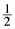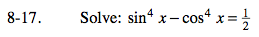### Home > PC > Chapter 8 > Lesson 8.1.1 > Problem8-17

8-17.

Solve: sin4x − cos4x =Homework Help ✎Factor.

Substitute using the Fundamental Pythagorean Identity.

Substitute using the Fundamental Pythagorean Identity.

Combine.

Divide both sides by −2.

Square root both sides. Don't forget the ±.

$\text{Draw a unit circle to find all angles whose cosine is }\pm \frac{1}{2}.$

$(\text{sin}^2x+\text{cos}^2x)(\text{sin}^2x-\text{cos}^2x)=\frac{1}{2}$

$(\text{sin}^2x-\text{cos}^2x)=\frac{1}{2}$

$1-\text{cos}^2x-\text{cos}^2x=\frac{1}{2}$

$-2\text{cos}^2x=-\frac{1}{2}$

$\text{cos}^2x=\frac{1}{4}$

$\cos x=\pm \frac{1}{2}$

$x=\pm \frac{\pi}{3}+\pi n$